# A Quick Kick Start on Pandas

Pandas library is vital in data science. data science is a process of analyzing a large set of data points to get answers to questions related to that data set. For that, Pandas is a python module that makes data science easy and effective. Pandas’ core is a data frame.

## Data Frame

Dataframe is the main object in Pandas. It is used to represent data with rows and columns. For a simple scenario, when you have data in the CSV file(spreadsheet), you have to represent the data in the Pandas. For that, you will load your CSV file data into the dataFrame object.

We will illustrate each example using the Jupiter notebook. We want data for that I have this spreadsheet file.

First We have to create a data frame object which will represent this data. We will use the read_csv method of pandas to create a dataframe object which can represent the above CSV file.

It looks like the same as the spreadsheet but you can do more things with this dataframe object.

you can use the info() method to get the details of the dataframe object

If you use the describe() method, it will give the statistics of each column

when you say shape, it will give the rows and columns in a tuple

We can get the first n rows using the head function as well we can get the last n rows using the tail function of the data frame

We can use the indexing and slicing here as well. when we need the second row to the fourth row we can use the slicing here.

When you need columns you can get the columns below and if you want to see the particular column then you can use the syntax like this dataframe.column_name or data_frame[‘column_name’]

We have some scenario like we have to take the two or three columns from our dataframe for that, we have to specify the column names inside [[]] here.

What are the operations we can do with this data set

1. We can get the maximum using the max method
2. We can get the mean using the mean method
3. We can get the median using the median method

We can select the rows with conditions. for example, we can query the data frame to give the rows where the area is greater than 3500 as below

from here, you can view all operations of pandas.

When you see here, the index is 0 to 5, How can we change this index? We can set one column as an index then we can get the row using the index.

For that, I will use the area as the index.

Now we can get the row using the area value

We can reset the index using rest_index()

## Reading and writing to the excel or CSV files

When we are reading the CSV file we can skip the rows or we can specifically say in which place our header is there

`df = pd.read_csv("areaPrize", skiprows=1) # below also samedf = pd.read_csv("areaPrize", header=1)`

When we don’t have the headers in the CSV file we can give the headers as below

`df = pd.read_csv("areaPrize.csv", names = ["area", "bedroom", "age", "town", "price"])`

When we want to read some like three rows from the CSV file we can use below the nrows argument

`df = pd.read_csv("areaPrize", nrows=3)`

When we have multiple representations for the not available values we have to make them into one representation for that we can use the na_values argument

`df = pd.read_csv("areaPrize", na_values=['not avalilabe","na", "whatever"])# Also we can use this method to convey the particular columndf = pd.read_csv("areaPrize", na_values={'area':['not avalial'],'age':['nan'],-1]})`

This will use when you want to clean up the mess data.

How to write back to CSV file

`df.to_csv("new.csv")`

But it also wrote index as well. if you want to get rid of this index and you need only area and age

`df.to_csv("new.csv", index = False, columns=['area','age'])`

## How we can handle the missing data using pandas

1. fillna() is a method to fill the missing values using different ways
2. interpolate() is a method to guess missing values using interpolation.
3. dropna() is a method to drop the rows with missing values

We can replace all the NaN values with some other values as below

`new_df = df.fillna(0)`

Here, you can see that all the NaN values are replaced by 0 but it is not good. If you look at the town column what do you mean by 0 so we have to give the NaN values for each column? We can do it using the dictionary.

In reality, what are zero areas you may do you put the values which had the previous value? Here, it will curry the previous value to the NaN value. we can do it backfill as well(bfill()). you can use the limit to set how many copies for one value

We can use the interpolate to make a better guess for the area

`new_df.area = df.area.interpolate()new_df.age = df.age.interpolate(method ="time")`

More details can be found here.

When we drop the values, we will use dropna() function

`new_df = df.dropna()`

We can give conditions like when it has all values then we drop the column

`new_df = df.dropna(how = 'all')`

We can give a threshold to keep the row if we have at least non NAN value 2 then it will keep the row

`new_df = df.dropna(thresh=2)`

## Other techniques to handle missing data

This is my current data set. In this data set first I set the day as the index

We can replace the particular value with replacing the function of pandas. I will replace the value with NumPy NaN value

We can replace the values based on a specific column as well

these two methods worked well.

## GroupBy Feature in Pandas

If you look at this data set, you will see the cities weather details. How to know what is the maximum temperature in Jaffna? It means we have to group the data with cities for that, pandas have some feature, we will see below

`g_object = df.grouby('city')`

g_object is a groupby object of dataframe. This groupby object has cities as key and their data frame as values. For example, we have ‘jaffna, ‘colombo, ‘trinco’ keys and their respective rows are integrated into dataframe object and this dataframe will be the value of that key.

For a specific group, you can use the get_group() function of that object and it is a dataframe

Here we are dividing our data into groups and apply some analytics later we have combined the results.

Now we first groupby city and inside the city, we have a plan to group by event

Thanks for reading this Blog!!! Hope you will enjoy it.

## More from Sivaram Rasathurai

Software Engineer — Sysco LABS

Love podcasts or audiobooks? Learn on the go with our new app.

## My Journey into Digital Manufacturing## How To Use Namespaces In PHP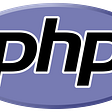## What constitutes a Web3 App or a dApp?## How to Create a DynamoDB Table Using Serverless Framework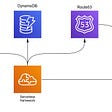## Business Backlog — Why you need one if you’re a product manager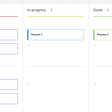## Core Values of Scaled Agile Framework® (SAFe)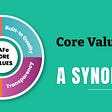## Exporting 2D drafting from Rhino to PDF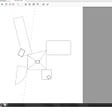## Introduction to Android Development- A new way of welcoming innovations!## Sivaram Rasathurai

Software Engineer — Sysco LABS

## Goalies Are Being Pulled Earlier in NHL; Does It Pay off?## Why is Mathematical Optimization Crucial for Every Data Scientist?## I need to learn data science from scratch, where do I start?